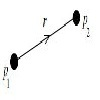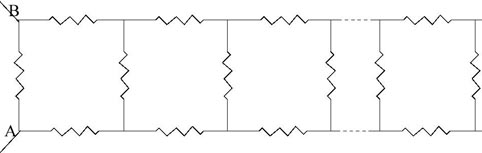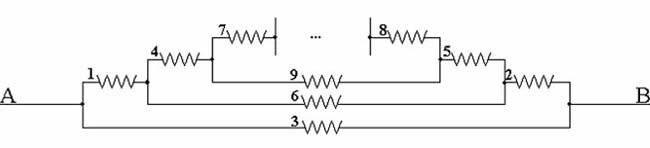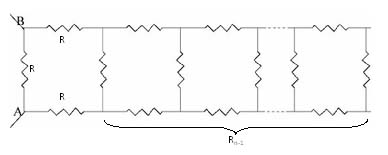#### You may also likeCan you arrange a set of charged particles so that none of them start to move when released from rest?

# Resistance

##### Age 16 to 18Challenge Level

Aleksander from Gdynia Bilingual High School No 3, Poland, David from Guilford County (is that UK, US or elsewhere?) Andrei from Romania and Chris from CSN used different methods to solve this problem, all of them leading to solving a quadratic equation. Chris used the observation that, removing three resistors leaves the resistance of the remaining network unchanged, and quickly arrived at the quadratic equation which gives the solution. Aleksander visualised the network in a different, but equivalent, configuration and used continued fractions and David's method was similar. Andrei arrived at the same quadratic equation by first proving that the sequence of resistances $R_0, R_1, R_2, ...$ from A to B, corresponding to different number of resistance 'squares', is a steadily decreasing sequence and, as $R_n > 0$, the sequence tends to a limit.

This is Chris's solution:

Following the hint, keep in mind that the equations for series resistances and parallel resistances are: $R = R_1 + R_2$ for series and $R = (R_1 \times R_2)/(R_1 + R_2)$ for parallel.The resistance $R$ between A and B is the equivalent of a resistance of 1 ohm in parallel with two resistances of 1 ohm and a resistance R in series and we can write the following equation: $$R = {1 \times (2 + R)\over 1 + (2 + R)} = {(2 + R)\over (3 + R)}.$$ Reworking the above equation we get: $R(3 + R) = (2 + R)$ or $$R^2 + 2R - 2 = 0.$$ Solving the quadratic equation we get two solutions, one of which we cannot use because it is negative, so $R = - 2.73205$ is not valid because a resistance cannot have a negative value. The correct solution is: $$R =\sqrt 3 -1 \approx 0.732051.$$

This is Aleksander's solution:

Generally it is easier to calculate the resistance of a circuit when you present it in a way that one end of circuit (here A) is on the left and the other is on the right. Here we have an illustration of our ladder circuit in such a way making it much easier to justify whether two resistors are in parallel or in series.:From the picture we know that resistors labeled 1, 2 and the group of resistors 4,5,6,7,8,9 (all 1 ohm) are connected in series. We can also observe that the resistor 3 is connected in parallel to the resistors mentioned before. Therefore, where $R$ is the resistance of the whole circuit, and $R_n$ is the resistance of the $n$th resistor, we can write: $$R = {1\over {1\over R_3} + {1\over R_1 + R_2 +...}}.$$ The three dots refer to the resistances of the resistors not mentioned in the formula, but occurring in the further part of the circuit.

We can observe that the pattern for the first three resistors repeats for the rest so $$R = {1\over {1\over R_3} + {1\over R_1 +R_2 + {1\over {1\over R_6} + {1\over R_4 +R_5+...}}}}$$ and the fraction grows like that to infinity. As all the resistances are 1 ohm this gives the continued fraction: $$R = {1\over 1 + {1\over 2 + {1\over 1 + {1\over 2 +...}}}} = {1\over 1 + {1\over 2 + R}}$$ After a few simplifications we obtain the quadratic equation : $R^2 + 2R - 2 = 0.$ As $R$ must be positive the solution is $R = \sqrt 3 - 1$ ohms.

This is Andrei's solution:

First I shall prove that the resistance from A to B tends to a limit as more 'squares' are added to the network. I shall denote the value of each resistance by $R$ and the values of the equivalent resistances between A and B, corresponding to different number of resistance 'squares', by $R_0, R_1, R_2, ...$ etc. Evidently $R_0 = R$ and $R_1 = 3R/4$.From the diagram I observe that: $${1\over R_n} = {1\over R} + {1\over 2R +R_{n-1}}.$$ where $R = 1$. I observe that $R_1< R_0$, and I shall prove by induction that $R_n< R_{n-1}$. This, by the recurrence relation, is equivalent to: $$R_n< R_{n-1}\Leftrightarrow {1\over R_n}> {1\over R_{n-1}} \Leftrightarrow {1\over R} + {1\over 2R + R_{n-1}} > {1\over R} + {1\over 2R + R_{n-2}}\Leftrightarrow R_{n-1} < R_{n-2}.$$ So, the sequence $(Rn)$ is strictly decreasing. Evidently $R_n > 0$ so the sequence $(R_n)$ is convergent. By considering the limit in the recurrence relation (putting $R=1$), $$\lim _{n\to \infty} R_n = {1\over {1+ {1\over 2 + \lim_{n\to \infty}R_{n-1}}}}$$ where $$\lim _{n\to \infty} R_n = \lim _{n\to \infty} R_{n-1} = R_{L}.$$ This gives $R_{L}= {2+ R_{L}\over 3 + R_{L}}$ and hence the quadratic equation $R_{L}^2 + 2R_{L} - 2= 0$. The positive solution of this equation gives the resistance of the network: $\sqrt 3 - 1$ ohms.